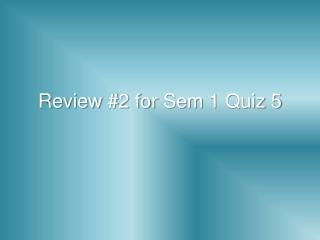DownloadDownload PresentationReview #2 for Sem 1 Quiz 5

# Review #2 for Sem 1 Quiz 5

Télécharger la présentation## Review #2 for Sem 1 Quiz 5

- - - - - - - - - - - - - - - - - - - - - - - - - - - E N D - - - - - - - - - - - - - - - - - - - - - - - - - - -
##### Presentation Transcript

1. Review #2 for Sem 1 Quiz 5

2. What conclusion can you draw? The doorbell rings. If the doorbell rings, then the dog will bark. The dog barks.

3. The sum of twice an angle and 3 times its complement is 220. Find the measure of the angle’s supplement. 2x + 270 – 3x = 220 -x = -50 x = 50 Supplement = 130° 2x + 3(90 – x) = 220

4. Write a biconditional for the statement below. If 2 angles are congruent, then they have the same measure Two angles are congruentiff they have the same measure.

5. Which quadrilaterals have: Parallelogram, rectangle, Rhombus Opposite angles congruent?

6. A D Is ADCB a valid name for the parallelogram? B C Yes!

7. C  ~h  u  ~m  z OR ~z  m  ~u  h  ~c Make a logic chain, and draw a conclusion. ~h  um  ~uc  ~h~m  z C  Z or~z  ~c

8. If the doorbell rings, then the dog will bark. What conclusion can you draw? The dog is barking. No conclusion!

9. A D Is DABC a valid name for the parallelogram? B C Yes!

10. W  ~m  q  l OR ~l  ~q  m  ~w Make a logic chain, and draw a conclusion. ~m  qm  ~wq  l W  l or~l  ~w

11. What conclusion can you draw? The doorbell has not rung. If the doorbell rings, then the dog will bark. No conclusion!

12. A D Is BDCA a valid name for the parallelogram? B C No!

13. Which quadrilaterals have: Rectangle & Isos Trapezoid Congruent diagonals?

14. Write a biconditional for the statement below. If a polygon has 3 sides, then it is a triangle. A polygon has 3 sides iff it is a triangle.

15. A  g  ~h  d  ~p OR P  ~d  h  ~g  ~a Make a logic chain, and draw a conclusion. A g~h  dp  ~dh  ~g A  ~P orp  a

16. Write a biconditional for the statement below. If 2 lines in a plane do not intersect, then they are parallel. 2 lines in a plane do not intersect iffthey are parallel.

17. A D Is BADC a valid name for the parallelogram? B C Yes!

18. What conclusion can you draw? The dog is quiet. If the doorbell rings, then the dog will bark. The doorbell has not rung.

19. Which quadrilaterals have: rectangle Perpendicular Sides?

20. A D Is BCAD a valid name for the parallelogram? B C No!

21. Which quadrilaterals have: rhombus 4 congruent Sides?

22. A D Is ACDB a valid name for the parallelogram? B C No!

23. The sum of an angle’s complement and its supplement is 210. find the measure of the angle’s supplement. 270 – 2x = 210 -2x = -60 x = 30 Supplement = 150° 90 – x + 180 – x = 210

24. Three times and angle, subtracted from the angle’s supplement is 100. Find the measure of the angle’s complement. -4x = -80 X = 20 complement = 160° 180 – x – 3x = 100

25. Which quadrilaterals have: Rhombus Perpendicular diagonals?

26. Which quadrilaterals have: Parallelogram, rectangle, rhombus Diagonals that bisect each other?

27. What conclusion can you draw? If the dog barks, the cat will hide. If the doorbell rings, then the dog will bark. If the doorbell rings, then the cat will hide.

28. A D Is ABCD a valid name for the parallelogram? B C Yes!

29. Write a biconditional for the statement below. If today is Friday, then tomorrow is Saturday. Today is Friday iff tomorrow is Saturday.

30. A D Is CBAD a valid name for the parallelogram? B C Yes!